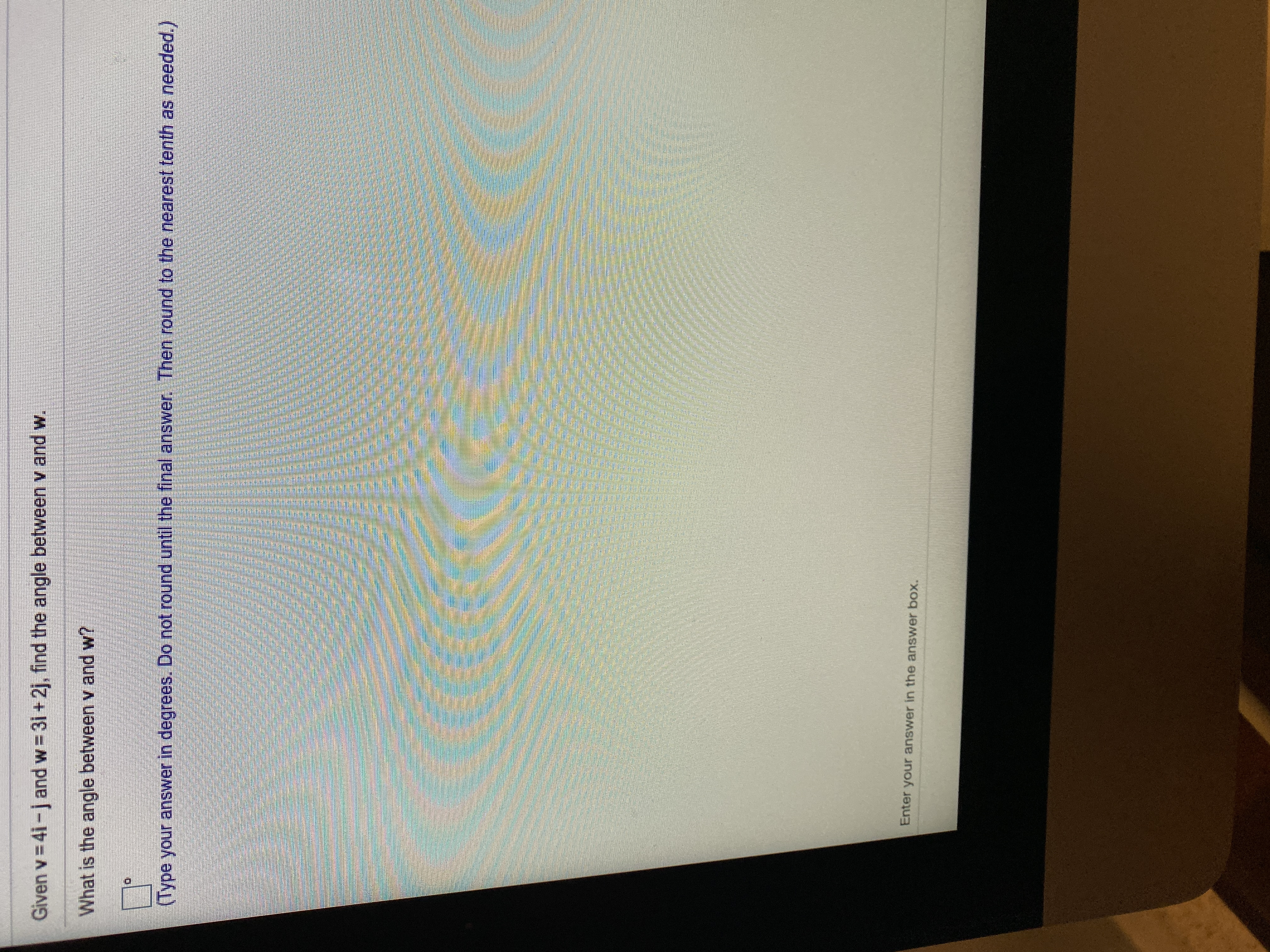# Given v 4i-jand w 3i+ 2j, find the angle between v and w.What is the angle between v and w?(Type your answer in degrees. Do not round until the final answer. Then round to the nearest tenth as needed.)Enter your answer in the answer box.

Question
6 views

practice questionshelp_outlineImage TranscriptioncloseGiven v 4i-jand w 3i+ 2j, find the angle between v and w. What is the angle between v and w? (Type your answer in degrees. Do not round until the final answer. Then round to the nearest tenth as needed.) Enter your answer in the answer box. fullscreen
check_circle

Step 1

To determine the angle between the vectors.

Step 2

Given:

v = 4i – j

w = 3i + 2j

Step 3

Formula Used:

Let a and b be two vectors and θ b...

### Want to see the full answer?

See Solution

#### Want to see this answer and more?

Solutions are written by subject experts who are available 24/7. Questions are typically answered within 1 hour.*

See Solution
*Response times may vary by subject and question.
Tagged in

### Other